How to Calculate and Solve for Rock Density Designation | Rock Mechanics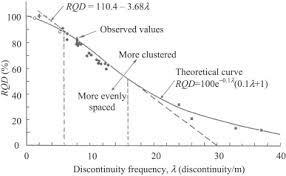The image above represents rock quality designation.

To compute for rock quality designation, two essential parameters are needed and these parameters are Sum of the Length of Core Pieces > 10cm (S) and Total Length Drilled or Core Recovery (L).

The formula for calculating the rock quality designation:

RQD = 100(S / L)

Where:

RQD = Rock Quality Designation
S = Sum of the Length of Core Pieces > 10cm
L = Total Length Drilled or Core Recovery

Let’s solve an example;
Calculate the rock quality designation? Given that the sum of the length of core pieces > 10cm is 20 and the total length drilled or core recovery is 14.

This implies that;

S = Sum of the Length of Core Pieces > 10cm = 20
L = Total Length Drilled or Core Recovery = 14

RQD = 100(S / L)
RQD = 100(20 / 14)
RQD = 100(1.428)
RQD = 142.85

Therefore, the rock quality designation is 142.85.

Calculating the Sum of the Length of Core Pieces > 10cm when the Rock Quality Designation and the Total Length Drilled or Core Recovery is Given.

S = RQD x L

Where:

S = Sum of the Length of Core Pieces > 10cm
RQD = Rock Quality Designation
L = Total Length Drilled or Core Recovery

Let’s solve an example;
Calculate the sum of the length of core pieces > 10cm? Given that the rock quality designation is 32 and the total length drilled or core recovery is 11.

This implies that;

RQD = Rock Quality Designation = 32
L = Total Length Drilled or Core Recovery = 11

S = RQD x L
S = 32 x 11
S = 352

Therefore, the sum of the length of core pieces > 10cm is 352.

Calculating the Total Length Drilled or Core Recovery when the Rock Quality Designation and the Sum of the Length of Core Pieces > 10cm is Given.

L = S / RQD

Where:

L = Total Length Drilled or Core Recovery
RQD = Rock Quality Designation
S = Sum of the Length of Core Pieces > 10cm

Let’s solve an example;
Calculate the total length drilled or core recovery? Given that the sum of the length of core pieces > 10cm is 26 and the rock quality designation is 42.

This implies that;

RQD = Rock Quality Designation = 42
S = Sum of the Length of Core Pieces > 10cm = 26

L = S / RQD
L = 26 / 42
L = 0.619

Therefore, the total length drilled or core recovery is 0.619.

Nickzom Calculator – The Calculator Encyclopedia is capable of calculating the rock quality designation.

To get the answer and workings of the rock quality designation using the Nickzom Calculator – The Calculator Encyclopedia. First, you need to obtain the app.

You can get this app via any of these means:

To get access to the professional version via web, you need to register and subscribe for NGN 1,500 per annum to have utter access to all functionalities.
You can also try the demo version via https://www.nickzom.org/calculator

Android (Paid) – https://play.google.com/store/apps/details?id=org.nickzom.nickzomcalculator
Android (Free) – https://play.google.com/store/apps/details?id=com.nickzom.nickzomcalculator
Apple (Paid) – https://itunes.apple.com/us/app/nickzom-calculator/id1331162702?mt=8
Once, you have obtained the calculator encyclopedia app, proceed to the Calculator Map, then click on Geology under Add-on.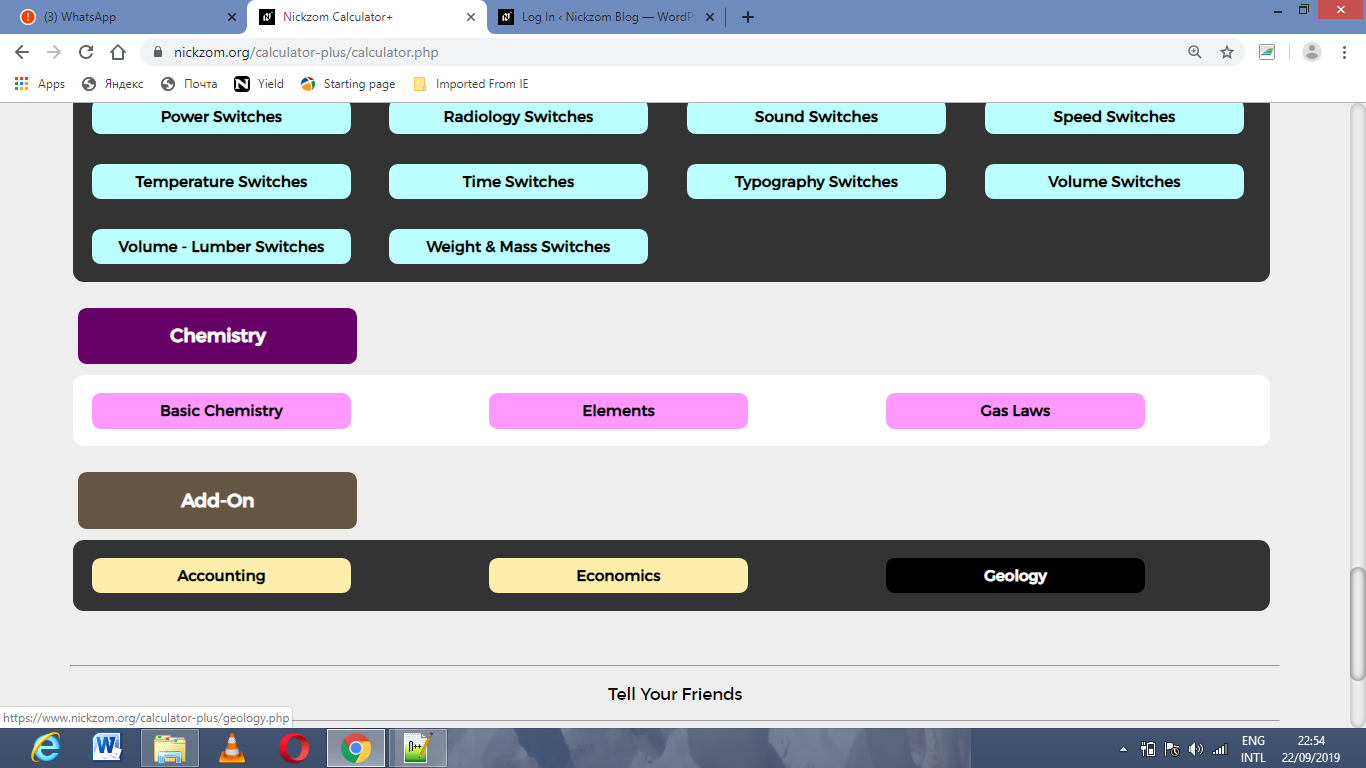Now, Click on Rock Mechanics under GeologyNow, Click on Rock Quality Designation under Rock Mechanics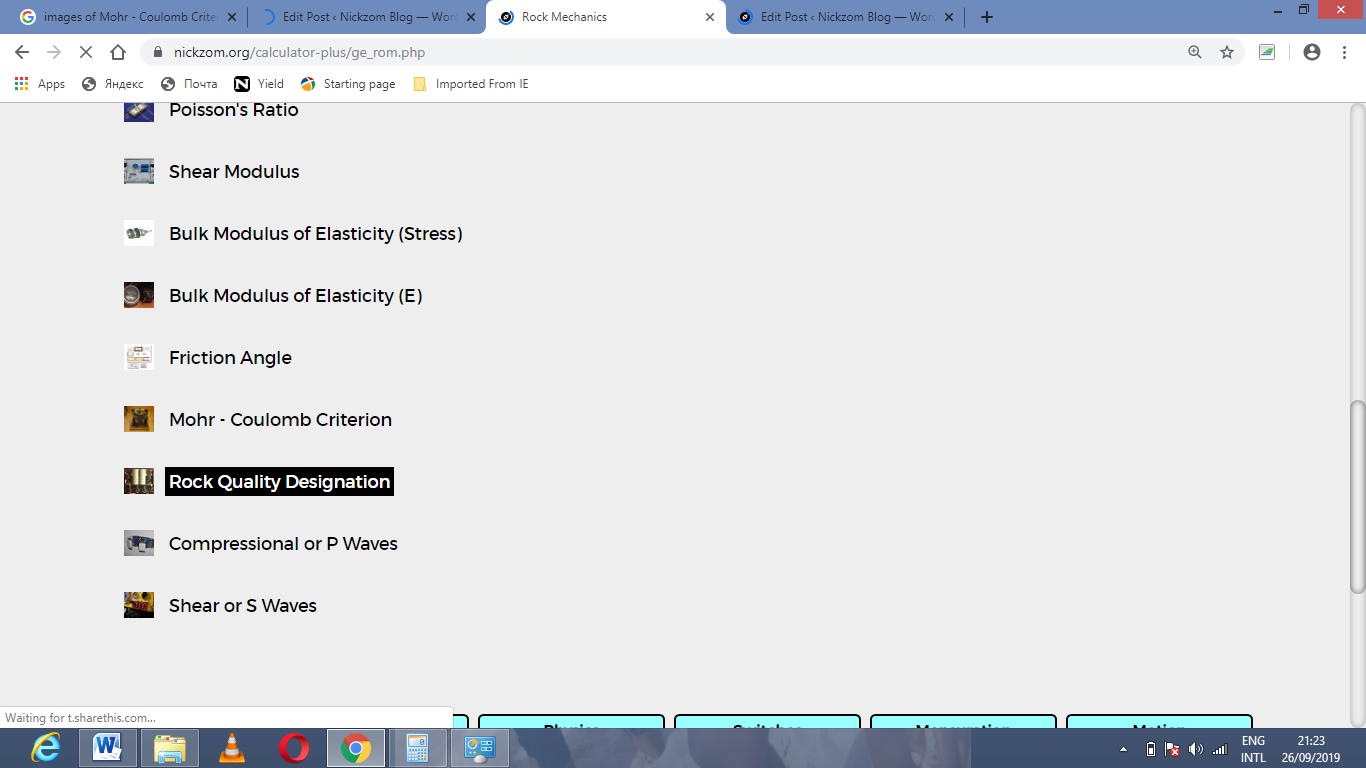The screenshot below displays the page or activity to enter your values, to get the answer for the rock quality designation according to the respective parameters which are the Sum of the Length of Core Pieces > 10cm (S) and Total Length Drilled or Core Recovery (L).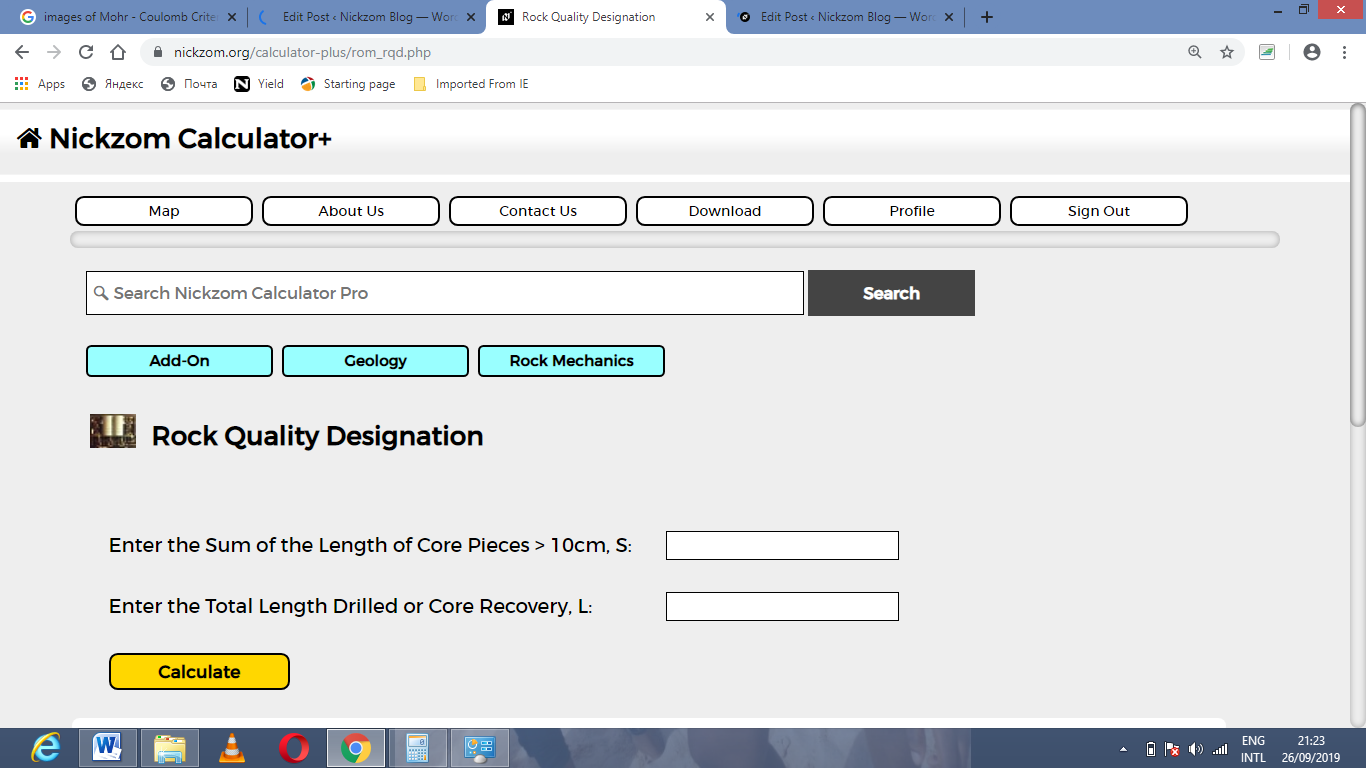Now, enter the values appropriately and accordingly for the parameters as required by the Sum of the Length of Core Pieces > 10cm (S) is 20 and Total Length Drilled or Core Recovery (L) is 14.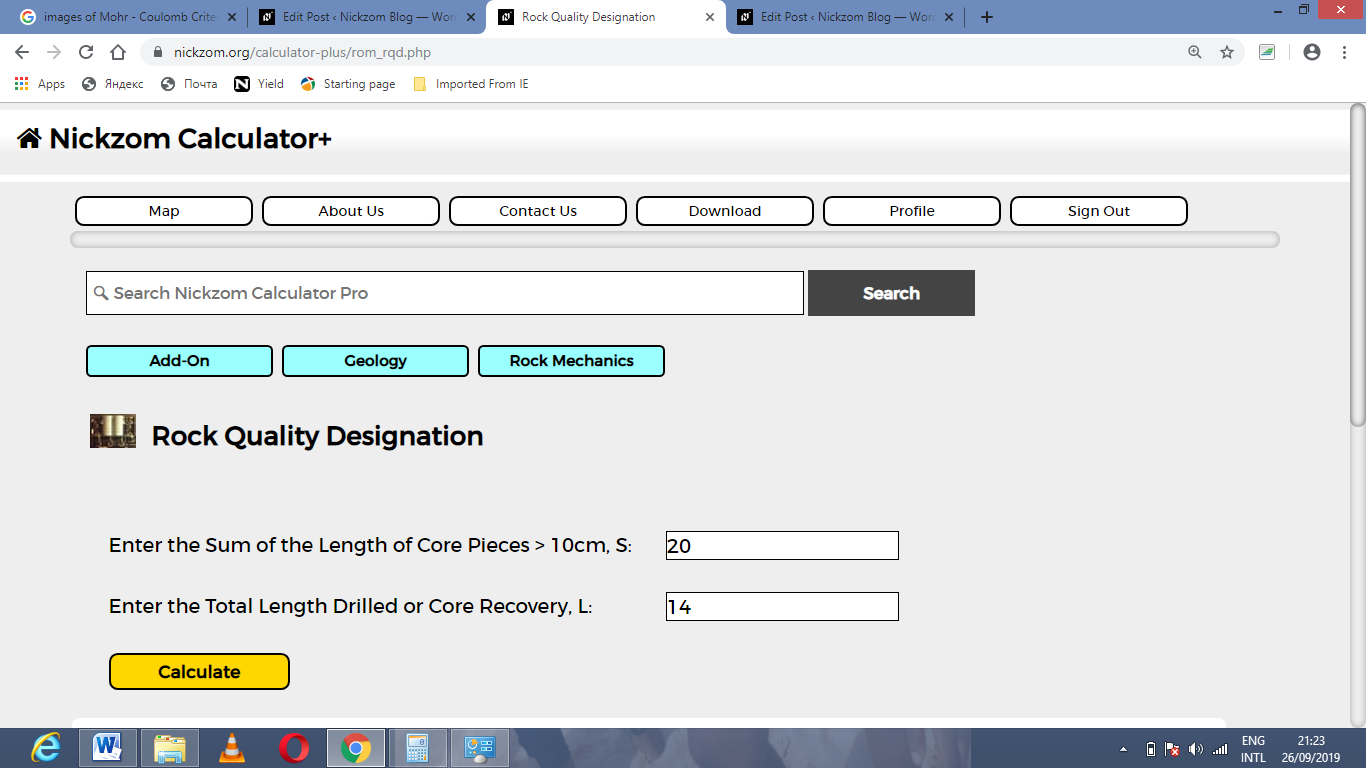Finally, Click on Calculate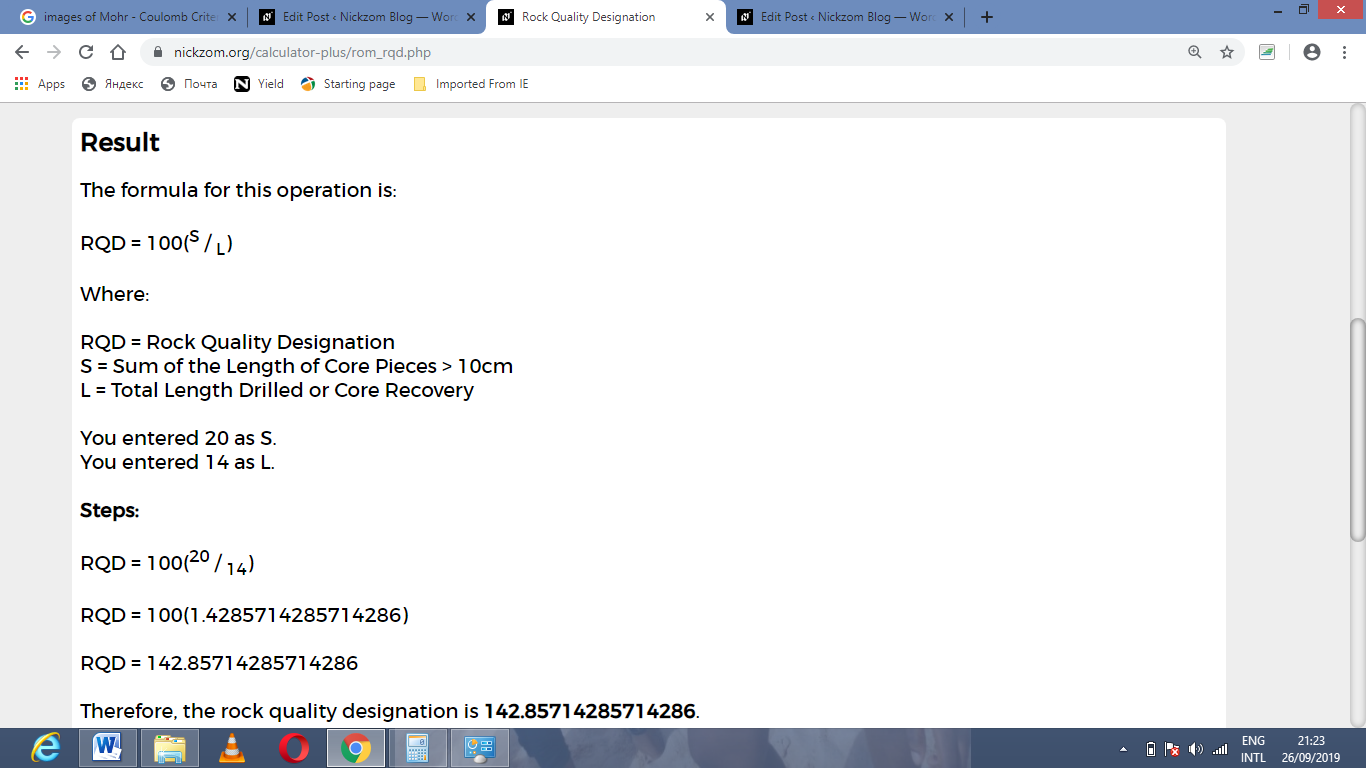As you can see from the screenshot above, Nickzom Calculator– The Calculator Encyclopedia solves for the rock quality designation and presents the formula, workings and steps too.